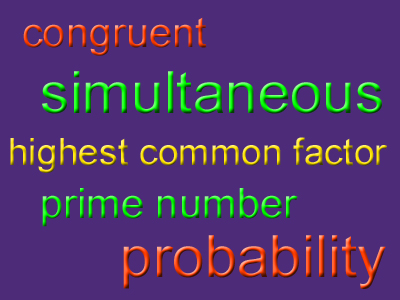# Mathematical Definitions 01 (F)

The world of mathematics could have a dictionary all to itself! This GCSE Maths quiz is the first of two that will test how well you know the meaning of some of the more commonly used words in maths. Try it for yourself and see how many you know!

Just as in other walks of life, when communicating maths it is important to be precise about what you are trying to describe. For instance, if I describe something as a rectangle, it suggests that the shape has 4 sides, the opposite sides are equal in length, and all the internal angles are right-angles. If my shape is missing any of these properties then it is not a rectangle, and I could mislead other people.

There are a number of words that we use in everyday life that have a different, or more precise meaning when used mathematically. Think of the word ‘similar’. In day-to-day language we use ‘similar’ to compare two objects that are alike in some ways, but not identical. However, in maths shapes can only be described as ‘similar’ if their corresponding sides are proportional to each other, AND corresponding angles are the same size. If we take the smaller shape, then each side is multiplied by the same scale factor to produce the enlargement, and all the angles stay the same.

Then there are those unusual words that only get used in maths, but not in the real world. CONGRUENT is one of these, can you think of any more? You still need to know what they mean, so best you start tucking into that maths dictionary!

Question 1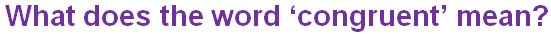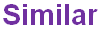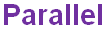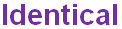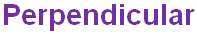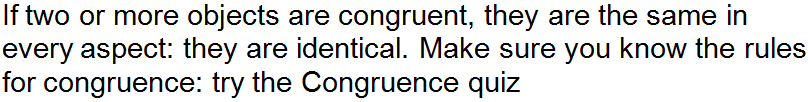Question 2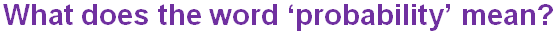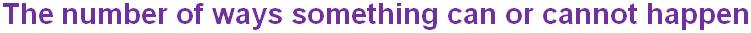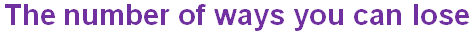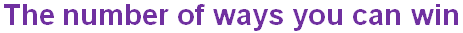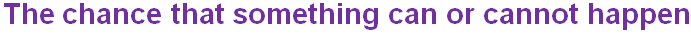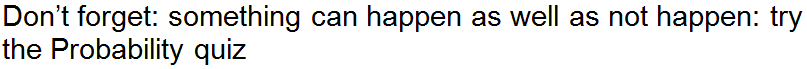Question 3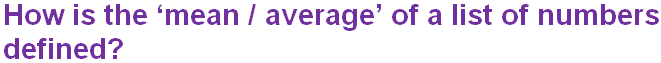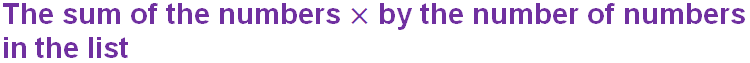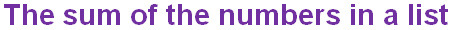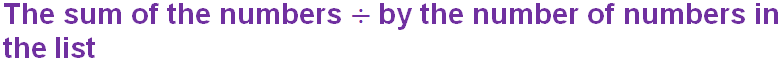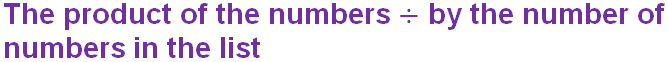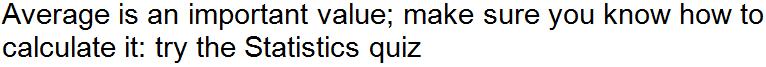Question 4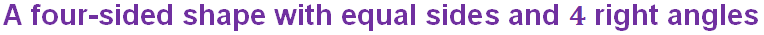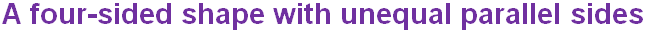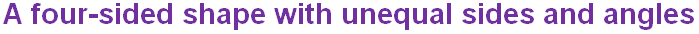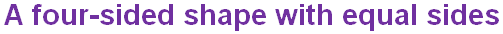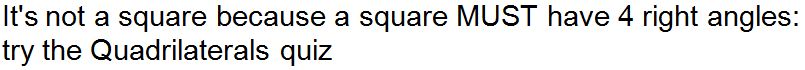Question 5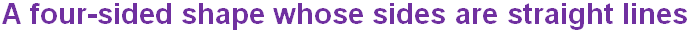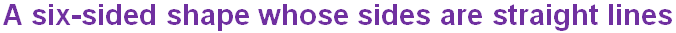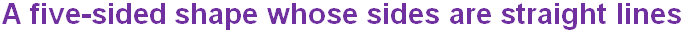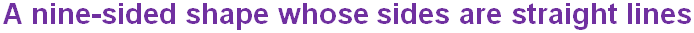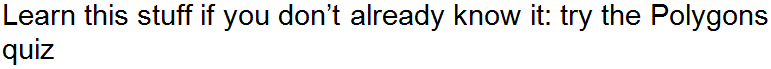Question 6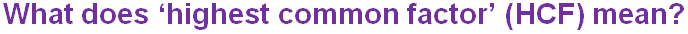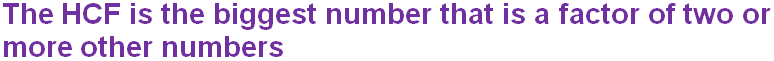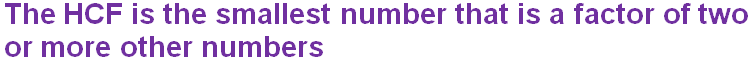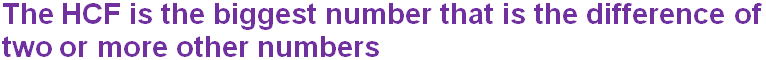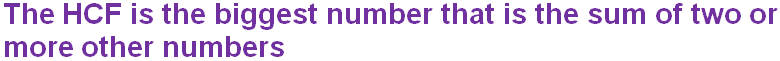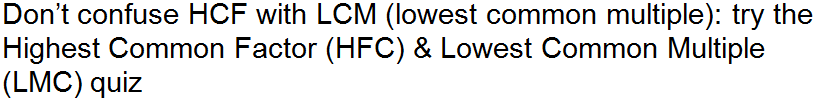Question 7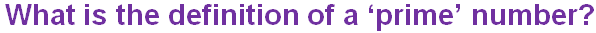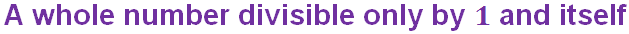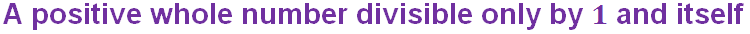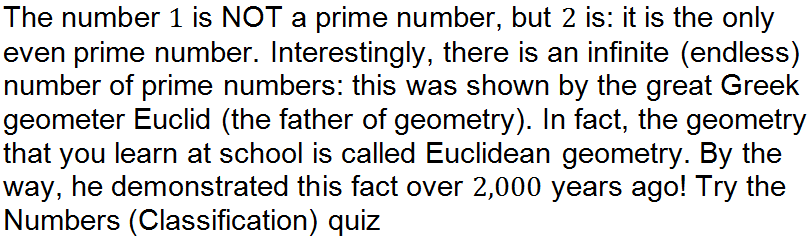Question 8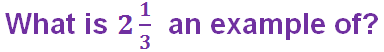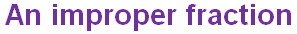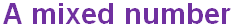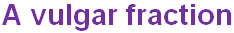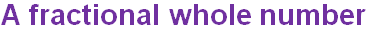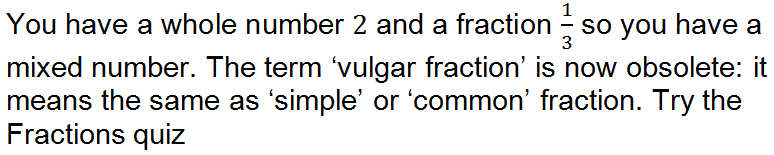Question 9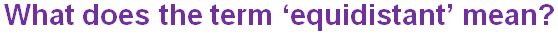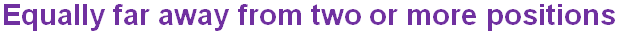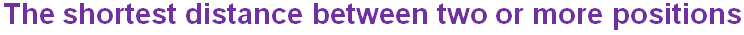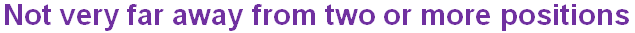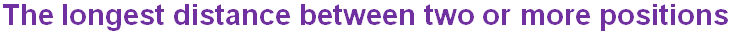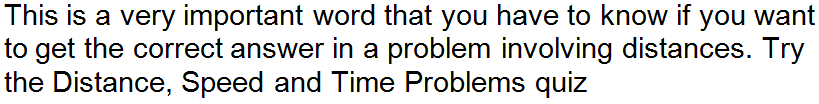Question 10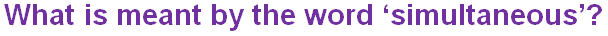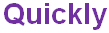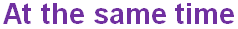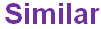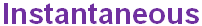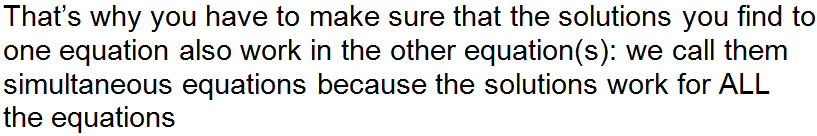Author:  Frank Evans